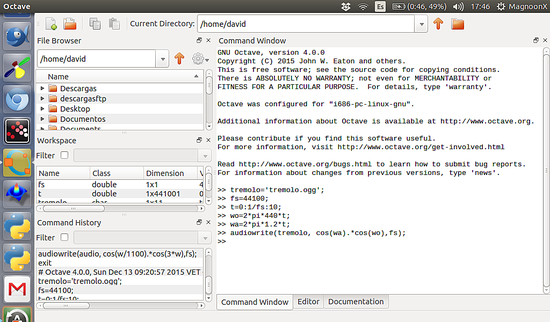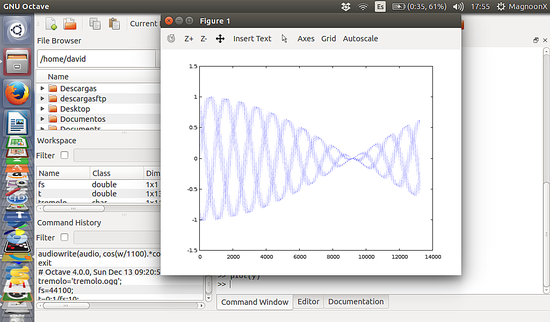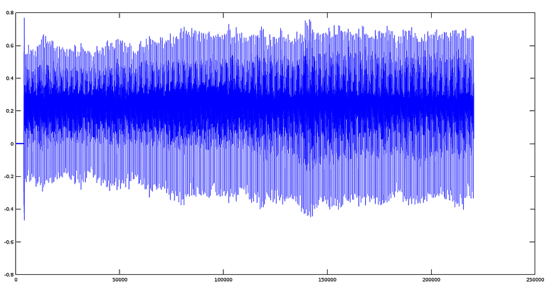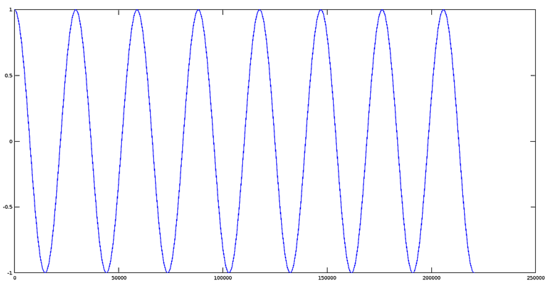# Scientific Audio Processing, Part III - How to apply Advanced Mathematical Processing Effects on Audio files with Octave 4.0 on Ubuntu

The third part of our Digital Audio processing tutorial series covers the signal Modulation, we explain how to apply Amplitude Modulation, Tremolo Effect, and Frequency Variation.

## Modulation

### Amplitude Modulation

As its name implies, this effect varies the amplitude of a sinusoid according to the message to be transmitted. A sine wave is called a carrier because it carries the information. This type of modulation is used in some commercial broadcasting and transmission citizen bands (AM).

### Why use the Amplitude Modulation?

If the communication channel is a free space, then antennas are required to radiate and receive the signal. It requires an efficient electromagnetic radiation antenna whose dimensions are of the same order of magnitude as the wavelength of the signal being radiated. Many signals, including audio components, have often 100 Hz or less. For these signals, it would be necessary to build antennas about 300 km in length if the signal were to be radiated directly. If signal modulation is used to print the message on a high-frequency carrier, let's say 100 MHz, then the antenna needs to have a length of over a meter (transverse length) only.

Concentration modulation or multi-channeling.

If more than one signal uses a single channel, modulation can be used for transferring different signals to different spectral positions allowing the receiver to select the desired signal. Applications that use concentration ("multiplexing") include telemetry data, stereo FM radio and long-distance telephony.

Modulation to Overcome Limitations on equipment.

The performance of signal processing devices such as filters and amplifiers, and the ease with which these devices can be constructed, depends on the situation of the signal in the frequency domain and the relationship between the higher frequency and low signal. Modulation can be used to transfer the signal to a position in the frequency domain where design requirements are met easier. The modulation can also be used to convert a "broadband signal" (a signal for which the ratio between the highest and lowest frequency is large) into a sign of "narrow band".

Audio Effects

Many audio effects use amplitude modulation due to the striking and ease with which it can handle such signals. We can name a few such as tremolo, chorus, flanger, etc. This utility is where we focus in this tutorial series.

### Tremolo effect

The tremolo effect is one of the simplest applications of amplitude modulation, to achieve this effect, we have to vary (multiply) the audio signal by a periodic signal, either sinusoidal or otherwise.

`>> tremolo='tremolo.ogg';>> fs=44100;>> t=0:1/fs:10;>> wo=2*pi*440*t;>> wa=2*pi*1.2*t;>> audiowrite(tremolo, cos(wa).*cos(wo),fs);`This will generate a sinusoid-shaped signal which effect is like a 'tremolo'.### Tremolo on real Audio Files

Now we will show the tremolo effect in the real world, First, we use a file previously recorded by a male voice saying 'A'. The plot for this signal is the following:

`>> [y,fs]=audioread('A.ogg');>> plot(y);`Now we have to create an enveloping sinusoidal signal with the following parameters:

Amplitude = 1
Frequency= 1.5Hz
Phase = 0

`>> t=0:1/fs:4.99999999;>> t=t(:);>> w=2*pi*1.5*t;>> q=cos(w);>> plot(q);`

Note: when we create an array of values of the time, by default, this is created in the form of columns, ie, 1x220500 values. To multiply this set of values must transpose it in rows (220500x1). This is the t=t(:) commandWe will create a second ogg file which contains the resulting modulated signal:

`>> tremolo='tremolo.ogg';>> audiowrite(tremolo, q.*y,fs);`

## Frequency Variation

We can vary the frequency to obtain quite interesting musical effects such as distortion, sound effects for movies and games among others.

### Effect of sinusoidal frequency modulation

This is the code where the sinusoidal modulation frequency is shown, according to equation:

Y=Ac*Cos(wo*Cos(wo/k))

Where:

Ac = Amplitude

wo = fundamental frequency

k = scalar divisor

`>> fm='fm.ogg';>> fs=44100;>> t=0:1/fs:10;>> w=2*pi*442*t;>> audiowrite(fm, cos(cos(w/1500).*w), fs);>> [y,fs]=audioread('fm.ogg');>> figure (); plot (y);`

The plot of the signal is:You can use almost any type of periodic function as the frequency modulator. For this example, we only used a sine function here. Please feel free to experiment with changing the frequencies of the functions, mixing with other functions or change, even, the type of function.view as pdf |print

## 1 Comment(s)

Please register in our forum first to comment.